Vibroengineering PROCEDIA

Published: 19 October 2018

# Research on dynamic responses of incompletely water-filled cylindrical shell subjected to explosion impact

Fuyin Gao1
Chong Ji2
Ying Liu3
Liangyu Cheng4
Yang Yu5
1, 2, 3, 4, 5College of Field Engineering, The Army Engineering University of PLA, Nanjing, 210007, China
Corresponding Author:
Chong Ji
Views 48

#### Abstract

Numerical simulation on the deformations and damages of incompletely water-filled cylindrical shells subjected to explosion impacts were carried out. The Research shows that the degree of deformation and failure of the incompletely liquid-filled cylindrical shell is smaller than that of the empty cylindrical shell in the same condition. The dynamic response of the completely water-filled cylindrical shell subjected to 75gTNT explosion impact is the same with the one of partially water-filled cylindrical shell. The anti-explosion performance of the 95 % volume water-filled cylindrical shell subjected to 200gTNT explosion impact is better. The explosion direction greatly influenced the dynamic response of partially filled-water cylindrical shell. The dynamic response of a partially water-filled cylindrical shell is approximately the same as that of the fully water-filled cylindrical shell, when the explosion direction is on one side of the water. The results show that internal pressure of water will increase when subjecting to impact loading, the anti-blast ability of tube structure significantly enhanced.

#### Highlights

• Numerical simulation on the deformations and damages of incompletely water-filled cylindrical shells subjected to explosion impacts were carried out.
• The Research shows that the degree of deformation and failure of the incompletely liquid-filled cylindrical shell is smaller than that of the empty cylindrical shell in the same condition.
• The explosion direction greatly influenced the dynamic response of partially filled-water cylindrical shell.

## 1. Introduction

Oil and gas pipeline are conducive to the quick and efficient transportation of oil and gas products, which can meet the needs of different regions and consumption levels, and provide a guarantee for the strategic deployment of national energy security. Once the oil and gas pipeline damaged, it will cause huge economic losses. Therefore, we must make clear the dynamic behavior of oil and gas pipelines subjected to explosion impact loading. Previous studies have focused on the dynamic response of thin-walled cylindrical shells subjected to explosive shock loading, like W. J. Schuman Jr , Chung Kim Yuen  et al., but few studies have focused on the deformation and failure of liquid-filled cylindrical shells. The liquid-filled medium in the pipeline can absorb part of the explosive shock energy, which can hinder the deformation of the pipeline. Nishida M. et al.  investigated that the diameter of the plug of the water-filled tube was slightly larger than one of the empty tube. But Qasim H. Shah et al.  considered that deformations of the water-filled pipes were smaller, compared with deformations of the empty pipes under impacts. Finite element method can solve the dynamic response of cylindrical shell structure by Prinja Nawal K. , it can analyze effectively the deformation and failure of water-filled cylindrical shell.

In the present paper, the numerical simulation on incompletely water-filled cylindrical shells of 2.75 mm thickness subjected to explosion impact by 75gTNT charge and 200gTNT charge with different stand-off distances were carried out. It focused mainly on the anti-explosion performance of the filling medium (water), TNT charge mass.

## 2. Numerical simulation

### 2.1. The calculation model

The calculation model involves in water-filled cylindrical shell, TNT charge and air. The model of metallic cylindrical shell adopted the purely Lagrangian, others adopted ALE. The mesh size of the air, water, TNT charge and stent is 0.25 cm. The mesh size of the metal cylindrical shell from midpoint to 10 cm away from midpoint is 0.125 cm, the other of which is 0.5 cm. A quarter of model was established, which adopted SOLID164 solid element and g-cm-μs unit, as showed in Fig. 1.

Fig. 1The calculation model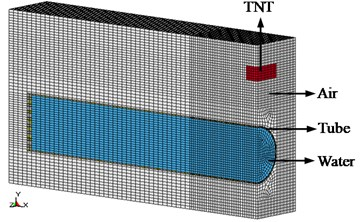### 2.2. The material parameter

The TNT model used Jones-Wilkens-Lee (JWL) equation of state (EOS) models, which engineering calculations is:

1
$p=\frac{{\rho }_{0}{c}^{2}\mu \left[1+\left(1-{\gamma }_{0}/2\right)\mu -a{\mu }^{2}/2\right]}{\left[1-\left({S}_{1}-1\right)\mu -{S}_{2}{\mu }^{2}/\left(\mu +1\right)-{S}_{3}{\mu }^{3}/{\left(\mu +1\right)}^{2}\right]}+\left({\gamma }_{0}+\alpha \mu \right)E.$

The state parameters are: ${\rho }_{e}=$ 1.63 g/cm3, $D=$ 6.93 km/s, ${p}_{CJ}=$ 21.1 GPa, ${A}_{1}=$ 371.1 GPa, ${B}_{1}=$ 3.230 GPa, ${R}_{1}=$ 4.16, ${R}_{2}=$ 0.96, $\omega =$ 0.30, ${E}_{0}=$ 7.0×109J/m3.

The air modeled used the simplest forms of EOS. The expression of pressure is:

2
$p=\left(\gamma -1\right)\rho e,$

where the air density $\rho$ is 1.225 kg/m3, $\gamma =$ 1.4, $e=$ 2.068×105 kJ/kg.

The water model adopted *MAT_NULL*. Where ${\rho }_{0}=$ 1000.0 kg/m3, ${\gamma }_{0}=$ 0.50.

The tube steel model adopted the Johnson -Cook material model , it defines the ${\sigma }_{y}$ is:

3
${\sigma }_{y}=\left[A+B\left({\stackrel{-}{\epsilon }}^{p}{\right)}^{n}\right]\left[1+C\mathrm{l}\mathrm{n}{\stackrel{˙}{\epsilon }}^{\mathrm{*}}\right]\left[1-\left(T\mathrm{*}{\right)}^{m}].$

The expression of effective fracture strain defined by:

4
${\epsilon }_{f}-\left[{D}_{1}+{D}_{2}\mathrm{e}\mathrm{x}\mathrm{p}{D}_{3}{\sigma }^{*}\right]\left[1+{D}_{4}\mathrm{l}\mathrm{n}{\stackrel{˙}{\epsilon }}^{*}\right]\left[1+{D}_{5}{T}^{*}\right].$

The material constants of the typical metal cylindrical shell are: $\rho =$ 7850 kg/m3, $\mu =$ 0.3, ${E}_{p}=$ 210 GPa, $A=$ 560 MPa, $B=$ 560 MPa, $n=$ 0.48.

## 3. Numerical analysis

The ALE fluid-solid coupling model has shown that it is possible to analyze the dynamic response of fully filled-water cylindrical shells subjected to blast loading, but it does not consider the deformation and damage of partially filled-water cylindrical shells. In fact, a liquid-filled pipe subjected to an explosion loading may also be not filled full water, because of the presence of the air inside the tube. The part-liquid cylindrical shell will show some different characteristics, and the air inside the tube provides the movement space for the liquid in the pipe. The dynamic response of partially filled cylindrical shells subjected to explosion impact will be different compared with that of full charging pipe.

Fig. 2The deformation process of the 2.75 mm thickness water-filled cylindrical shell under the 95 % filling volume subjected to 75gTNT charge explosion impacting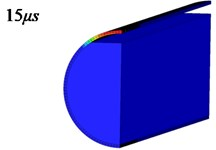a)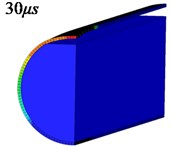b)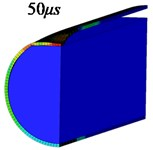c)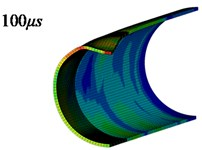d)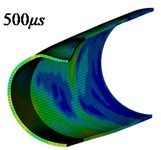e)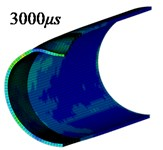f)

Fig. 3The deformation process of the 2.75 mm thickness water-filled cylindrical shell under the 85 % filling volume subjected to 200gTNT charge explosion impacting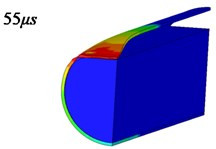a)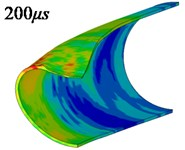b)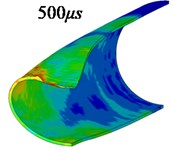c)

Fig. 2 is the deformation process of the 2.75 mm thickness water-filled cylindrical shell under the 95 % filling volume subjected to explosion impact of 75gTNT charge. Different from the fully water-filled cylindrical shell, the first interaction between the shell and the shell at 30 s is both sides of the horizontal plane. At the time of 50 s, the central point of the explosion surface is collided with the water medium, and the plastic hinge formed on both sides of the surface of the water medium plane. At 100 s, the sunken area continues to deform along the plastic hinge, the plastic hinge is prominent, and the shell strain changes. The maximum strain near the center of the explosion surface reaches 42.2 %. At the same conditions, the forming time of plastic hinge of empty tube is later, and is much smooth, and it will not form a convex shape. The maximum strain near the center point of the explosive surface is only 19.2 %. The deformation of the cylindrical shell structure is stable at 500 s, and the depth of the sunken pit is smaller than that of the empty tube, but the radial deformation area is larger. As shown in Fig. 3, when the distance between 200gTNT and 9 cm is 55 s, the center of the explosion surface will collide with the water medium. At this time, the two sides contacting with the water medium plane also form plastic hinge and form a sharper convex shape. The highly speed collision between the shell and the water medium, which is coupled with the water medium Poisson, together to absorb the blast impact energy. Due to the continuous interaction with the water medium, the strain in the sunken area increases gradually, and the shell near the center of the blasting surface becomes very thin. The maximum strain even reaches 69 %, which is far beyond the limit rupture strain of the steel itself. It can be seen that the ultimate fracture strain of the cylindrical shell is increased by the water-filled medium under the blast impact, and the impact toughness and strength of the cylindrical shell structure are improved.

The pressure values of the center point of partially filled cylindrical shells subjected to explosion impact show the regular trend, and the peak pressure peak of the center point is as shown in Fig. 4. After impacted by the shell, the peak of the secondary maximum pressure of the water medium is not the center point, but the water-filled volume is 95 %, and then the pressure peak does not fluctuate greatly. The larger end pressure peaks are 100 % water filled and 95 % water filled. It has intense pressure oscillation before 1000 μs in the end of 100 % water-filled cylindrical shell, after 1000 μs, the end of 95 % water-filled cylindrical shell start oscillation to 2000 μs. The end deformation is not consistent, because water shock pressure small end deformation is broadly in line with a final degree of transverse convex shape. The center peak pressure is 2.47 MPa under 200gTNT explosion and distance of 9 cm, but the end of the peak pressure in the 95 % and 85 % water-filled volume is more than 100 % water-filled volume. The pressure peak is in the 95 % water-filled volume, indicated strong oscillations and large deformation of the cylindrical shell, in the condition of the water-filled cylindrical shell water medium was more intense, the end deformation was severe.

Fig. 4The center and end pressure curve of a water filled cylindrical shell subjected to the 75gTNT explosion impact and 5cm explosion distance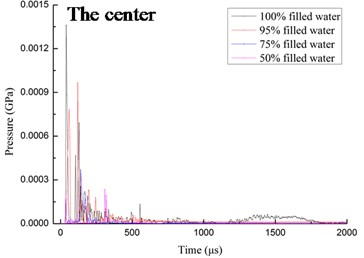a)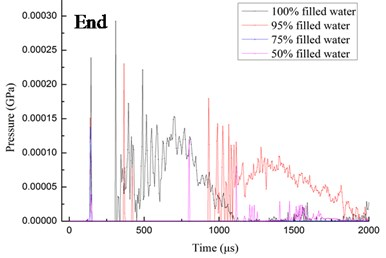b)

Fig. 6 is the radial and axial deformation curve of a water-filled cylindrical shell subjected to the 75gTNT charge and 5 cm explosion distance. The axial and radial deformation curves of the incomplete cylindrical shell are consistent with the trend from the figure, namely water filled more sunken, deformation is smaller. The sunken depth of the water 50 % filled volume is similar to the depth of empty tube, which is 5.7 cm and 5.78 cm respectively. The sunken curvature radius of the empty tube is only larger. It indicates that 75gTNT has no detonation effect in the 50 % volume under the 5 cm explosive distance. When the volume of water-filled reaches 95 % and 100 %, the radial curve of 100 % water-filled volume is larger when 95 % water-filled volume. Due to the intense collision between the water and the shell, the maximum strain near the center of the explosion surface reaches 42.2 % when the water-filled volume is 95 %, and the maximum strain is only 5.5 % when it is 100 %, the shell and the water become the whole movement.

As mentioned above, the empty tube subjected to 200gTNT explosion impact and 9 cm explosion distance will break through rupture; but when the water-filled volume reaches 75 %, the water-filled cylindrical shell will only have depression deformation and overall bending, as shown in Fig. 7. Compared with 75gTNT explosion impact, the sunken deformation degree is more serious, and the deformation regularity is different. When the water filling volume is 95 %, the sunken depth is the smallest, and the axial and radial deformation curves are uneven. It shows the violent collision degree between the shell and the water, resulting in the maximum strain of the shell is 57.3 %. When the water filling volume is 85 %, the plastic hinge appears near the center point of 3 cm, and is obviously convex and the axial deformation curve is not smooth. This is due to the collision between the shell and the water, and the maximum strain of the shell is 68.7 %. The axial and radial deformation curves of the 75 % and 100 % water-filled volumes tube are smoother, and the impact of the shells on the water is relatively low. The maximum strain of 75 % falls to 65 %, while the 100 % water-filled volume is only 13.4 %, the axial deformation area is the smallest.

Fig. 5The center and end pressure curve of a water filled cylindrical shell subjected to the 200gTNT explosion impact and 9 cm explosion distance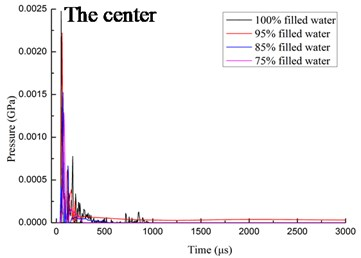a)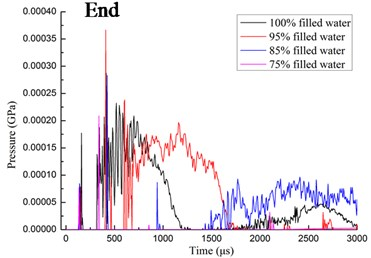b)

Fig. 6The radial and axial deformation curve of a water filled cylindrical shell subjected to the 75gTNT explosion impact and 5 cm explosion distance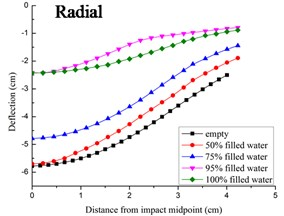a)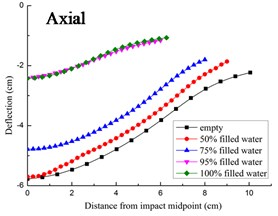b)

Fig. 7The radial and axial deformation curve of a water filled cylindrical shell subjected to the 200gTNT explosion impact and 9 cm explosion distance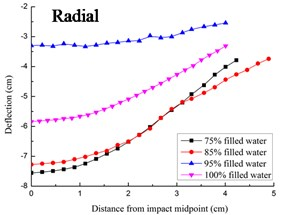a)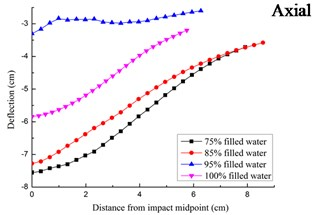b)

Except the water-filled volume affects antiknock performance of cylindrical shell, the explosion source orientation on anti-explosion performance of the incomplete water-filled cylindrical shell will also have an impact. Fig. 8 is the radial and axial deformation curve of a water filled cylindrical shell subjected to the 75gTNT front and inferior explosion impact and 5 cm explosion distance. It can be seen that the depression of the cylindrical shell under inferior explosion impact is smaller and close to the depressions of the fully water filled cylindrical shells. Therefore, Studying the antiknock performance of incomplete water filled cylindrical shells (water volume is greater than 50 %), if the explosion occurs inferior of partial water-filled cylindrical shells, it can be equivalent to the dynamic response of cylindrical shells with 100 % water-filled volume subjected to the impact of explosion.

Fig. 8The radial and axial deformation curve of a water-filled cylindrical shell subjected to the 75gTNT front and inferior explosion impact and 5 cm explosion distance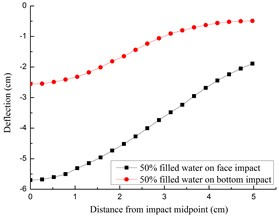a)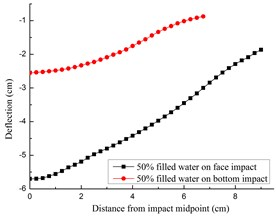b)

## 4. Conclusions

1) The pressure of the water increased by the joint action of the explosion impact and the deformation of the shell, which causes the Poisson coupling between the water and the cylindrical shell. Because of the presence of air in the pipe, when the impact is on the cylindrical shell, the air in the pipe provides the movement space for the liquid in the pipe. The degree of deformation and failure of the incompletely filled-water cylindrical shell is smaller than that of the empty pipe in the same condition;

2) The dynamic response of the fully water-filled cylindrical shell subjected to 75gTNT explosion impact is the same as that of incompletely water-filled cylindrical shell. The anti-explosion performance of the 95 % volume water-filled cylindrical shell subjected to 200gTNT explosion impact is better. The explosion direction greatly influenced the dynamic response of partially filled-water cylindrical shell. The dynamic response of the incompletely water-filled cylindrical shell is approximately the same as that of the fully water-filled cylindrical shell when the explosion direction is on one side of the water.

#### Acknowledgements

This research was financially supported by the National Nature Science Foundation of China, Nos. 51678567, 11102233 and 51608530.

#### Cited by

A theoretical modelling study on deep buried pipelines subjected to blast-induced ground shock